Computational & Technology Resources an online resource for computational,engineering & technology publications not logged in - login Civil-Comp ProceedingsISSN 1759-3433 CCP: 83PROCEEDINGS OF THE EIGHTH INTERNATIONAL CONFERENCE ON COMPUTATIONAL STRUCTURES TECHNOLOGY Edited by: B.H.V. Topping, G. Montero and R. Montenegro Paper 169An Iterative Radial Simplex Method for Elastostatic and Elastodynamic Boundary Elements K. Davey and M.T. Alonso RasgadoSchool of Mechanical, Aerospace and Civil Engineering, The University of Manchester, United Kingdom doi:10.4203/ccp.83.169 Full Bibliographic Reference for this paper K. Davey, M.T. Alonso Rasgado, "An Iterative Radial Simplex Method for Elastostatic and Elastodynamic Boundary Elements", in B.H.V. Topping, G. Montero, R. Montenegro, (Editors), "Proceedings of the Eighth International Conference on Computational Structures Technology", Civil-Comp Press, Stirlingshire, UK, Paper 169, 2006. doi:10.4203/ccp.83.169 Keywords: integration, simplexes, boundary elements, elastodynamics. Summary In this paper the iterative radial simplex method is introduced for the evaluation of singular integrals in boundary elements. The method involves the careful employment of multiple integration where the inner integral is performed along a radial direction. Evaluation of the radial-inner integral on a simplex of dimension n provides n+1 integrals on simplex domains of dimension n-1. On each simplex in the lower dimension the procedure is repeated and so on until the final dimension is 0 which yields an analytical solution or the singularity is sufficiently remote to facilitate numerical integration. Attention in the paper is restricted to dimensions 3, 2 and 1 with integration performed on tetrahedrons, triangles and closed intervals. In addition the super-singular integral equation is obtained from the differentiation of the hyper-singular integral equation. The super-singular integral equation has been developed for the purpose of analysing surface waves, which requires continuity of inter-element stresses, which is not present with the standard forms of boundary elements. Illustrated in the paper through detailed examples is the method's ability to evaluate to high accuracy the severe singular integrals involved in elastostatics and elastodynamics. The boundary element method (BEM) offers distinct advantages over domain methods such as the finite element method (FEM), one being the avoidance of domain discretisation. The avoidance of domain integration is achieved with the use of Green's functions, which are solutions to the governing equations for the case of an instantaneous source applied in an infinite or semi-infinite domain. The embodiment of these analytical solutions in the governing integral equations provides the boundary element method with high stability and accuracy that is often lacking with other competing methods. The use of Green's function offers advantages as well as disadvantages in that they can be complex in form and are singular making their integration problematic. Discussed in this paper is a method utilising domain integration. Although this could be viewed as a retrograde step, the advantages can outweigh the disadvantages. The background mathematics underpinning the method requires the concepts of solid angle and spherical co-ordinates in an Euclidean space of dimensions 3, 2 and 1. A point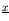in a real Euclidean space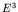classically takes the form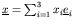, where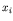are co-ordinate functions. Introduced in the paper is the spherical system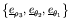of orthogonal unit vectors with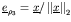, such that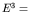span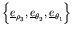. The Jacobean matrix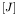for the mapping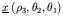is obtainable on differentiation of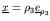with respect to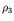,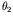and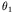. It can be shown that the Jacobean matrix is of the form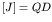, where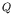is an orthogonal matrix and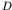is a diagonal matrix. Standard results of advanced calculus can be employed to represent the volume 3-form d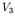as d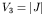ddd, where the Jacobean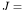det. Radial integration theory for non-singular and singular integrals on non-convex domains is presented in the paper. This involves application of the formula d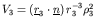dd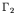, whereis the boundary for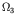,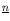is the outward pointing normal on,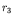is the radial distance from the origin to the 2-form d. The formula of interest is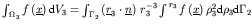, which is shown to be applicable to non-convex domains. In addition, the recursive formula of principal interest is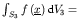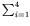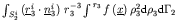, where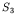is an 3-dimensional simplex (tetrahedron), whilst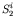are 2-dimensional simplexes (triangles) whose union forms the boundary of. To demonstrate the application of the recursive integration scheme to the elastodynamics, BEM integration over a 2-simplex is investigated. The simplex utilised is the triangular element with nodal co-ordinates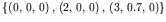. The results for the source point placed at various distances above the element edge and at frequency of 20,000 rad/s are tabulated in the paper. It is apparent that high accuracy is best achieved with the recursive method. Described in the paper is a recursive integration method that can be employed to evaluate, to high accuracy, singular boundary and domain integrals on simplex element domains. The following conclusions can be made: (i) The scheme provides arbitrary accuracy irrespective of the closeness of the source point to the element. (ii) The scheme is computationally more demanding than standard approaches but can be used when accuracy is a issue. purchase the full-text of this paper (price £20) Back to top ©Civil-Comp Limited 2023 - terms & conditions